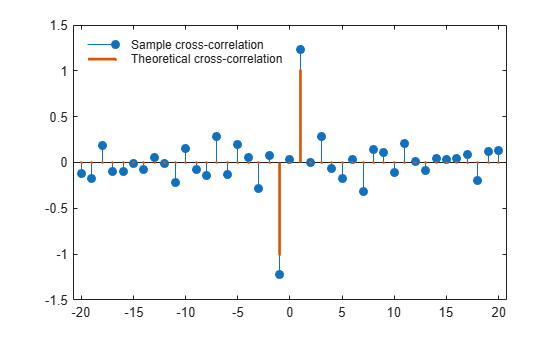Documentation

## Cross-Correlation of Two Moving Average Processes

This example shows how to find and plot the cross-correlation sequence between two moving average processes. The example compares the sample cross-correlation with the theoretical cross-correlation. Filter an $N\left(0,1\right)$ white noise input with two different moving average filters. Plot the sample and theoretical cross-correlation sequences.

Create an $N\left(0,1\right)$ white noise sequence. Set the random number generator to the default settings for reproducible results. Create two moving average filters. One filter has impulse response $\delta \left(n\right)+\delta \left(n-1\right)$. The other filter has impulse response $\delta \left(n\right)-\delta \left(n-1\right)$.

```rng default w = randn(100,1); x = filter([1 1],1,w); y = filter([1 -1],1,w);```

Obtain the sample cross-correlation sequence up to lag 20. Plot the sample cross-correlation along with the theoretical cross-correlation.

```[xc,lags] = xcorr(x,y,20,'biased'); Xc = zeros(size(xc)); Xc(20) = -1; Xc(22) = 1; stem(lags,xc,'filled') hold on stem(lags,Xc,'.','linewidth',2) q = legend('Sample cross-correlation','Theoretical cross-correlation'); q.Location = 'NorthWest'; q.FontSize = 9; q.Box = 'off';```The theoretical cross-correlation is $-1$ at lag $-1$, $1$ at lag $1$, and zero at all other lags. The sample cross-correlation sequence approximates the theoretical cross-correlation.

As expected, there is not perfect agreement between the theoretical cross-correlation and sample cross-correlation. The sample cross-correlation does accurately represent both the sign and magnitude of the theoretical cross-correlation sequence values at lag $-1$ and lag $1$.